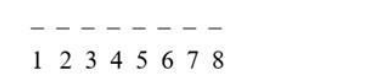# It is required to seat 5 men and 3 women in a row so that the women occupy

Question:

It is required to seat 5 men and 3 women in a row so that the women occupy the even places. How many such arrangements are possible?

Solution:

To find: number of arrangements in which women sit in even places

Condition: women occupy even places

Here the total number of people is 8 .In this question first, the arrangement of women is required.

The positions where women can be made to sit is $2^{\text {nd }}, 4^{\text {th }}, 6^{\text {th }}, 8^{\text {th }}$. There are 4 even places in which 3 women are to be arranged.

Women can be placed in $P(4,3)$ ways. The rest 5 men can be arranged in $5 !$ ways.

Therefore, the total number of arrangements is $P(4,3) \times 5 !$

Formula:

Number of permutations of $n$ distinct objects among $r$ different places, where repetition is not allowed, is

$P(n, r)=n ! /(n-r) !$

Therefore, a permutation of 4 different objects in 3 places and the arrangement of 5 men are

$P(4,3) \times 5 !=\frac{4 !}{(4-3) !} \times 5 !$

$=\frac{24}{1} \times 120$

= 2880.

Hence number of ways in which they can be seated is 2880.